# Friction Force Matrices

Let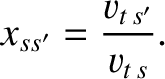(A.24)

In the following, all quantities that are of order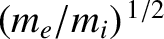,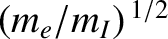, or smaller, are neglected with respect to unity. The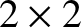dimensionless ion collisional friction force matrices,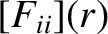,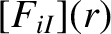,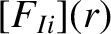, and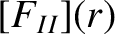, are defined to have the following elements (see Section 2.16) [7,9]: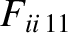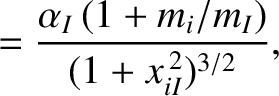(A.25)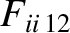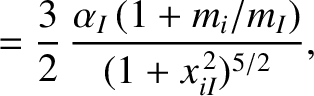(A.26)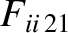(A.27)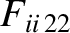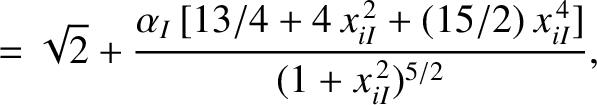(A.28)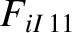(A.29)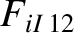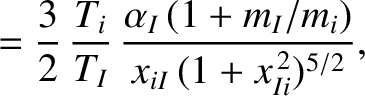(A.30)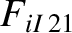(A.31)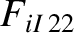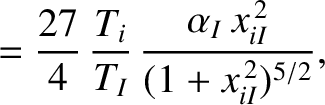(A.32)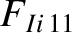(A.33)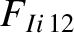(A.34)(A.35)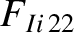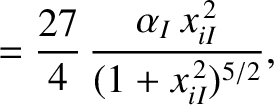(A.36)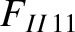(A.37)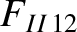(A.38)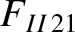(A.39)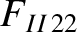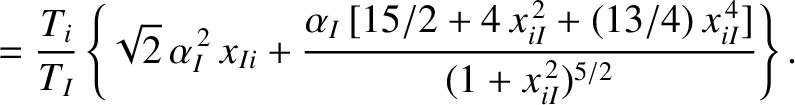(A.40)

Thedimensionless electron collisional friction force matrices,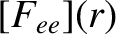,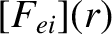, and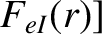are defined to have the following elements (see Section 2.16) [7,9]: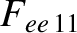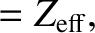(A.41)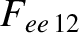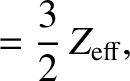(A.42)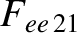(A.43)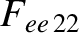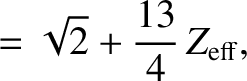(A.44)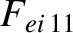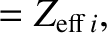(A.45)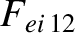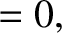(A.46)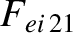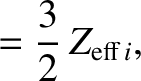(A.47)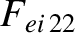(A.48)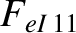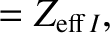(A.49)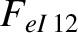(A.50)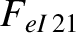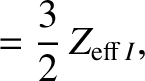(A.51)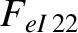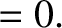(A.52)